Next: Exercises Up: One-Dimensional Compressible Inviscid Flow Previous: Piston-Generated Shock Wave

# Piston-Generated Expansion Wave

Consider the situation illustrated in Figure 14.3 in which, at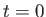, a tight-fitting piston is suddenly withdrawn from a stationary gas, contained in a uniform tube, at the steady speed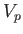, generating an expansion wave that propagates away from the piston, and into the gas. Suppose that the piston is located at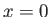at, and moves in the-direction. As described in Section 14.4, the expansion wave is isentropic.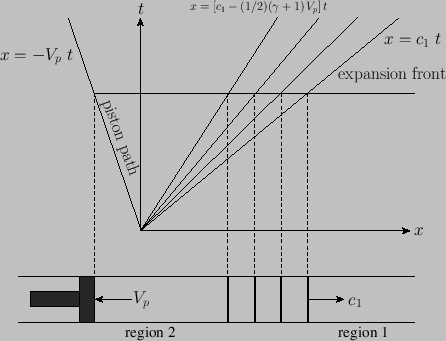The sudden withdrawal of the piston creates a step-function change in the flow speed, pressure, density, and temperature, of the gas inside the tube, such that the immediately adjacent gas moves with the piston. As described in Section 14.4, this step function flattens as the expansion wave begins to propagate. Locally, within the wave, the disturbance travels at the sound speed. Because the temperature varies across the wave (which starts out as a step in this variable), the local speed of disturbance propagation also varies across the wave. On the edge of the wave adjacent to the region of undisturbed gas, which we shall denote region, the temperature and propagation speed are greatest. On the opposite edge, the temperature and propagation speed are least. Let the region lying between the piston and the left edge of the expansion wave be denoted region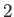. In region, the flow speed, pressure, density, and temperature, of the gas are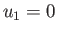,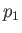,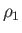, and, respectively. On the other hand, in region, the flow speed, pressure, density, and temperature, of the gas are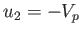(i.e., the gas in region 2 is co-moving with the piston),,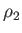, and, respectively. As shown in Figure 14.3, the expansion wave expands, or fans'' out, as it propagates. In fact, the-plot is a fan of constant sonic speed lines that show the development of the wave. These lines are called characteristics, and follow the path of local isentropic disturbances. The absolute speed of the disturbance is the sum of the local sonic speed and the local gas flow speed. The terminating characteristic on the right has slope, where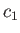is the region-sound speed. The terminating characteristic on the left has the slope, where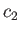is the region-sound speed. The latter slope may be either positive or negative, depending on whetheris greater than, or less than,.

Making use of isentropic relationships (see Section 14.3), we can explicitly evaluate the structure of the fan as follows. In terms of, the local sonic speed in the fan can be written [cf., Equation (14.47)]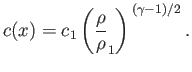(14.136)

Moreover, the relationship between the gas speed,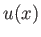, and the density in the wave is [cf., Equation (14.48)](14.137)

The previous two expressions yield [cf., Equation (14.50)]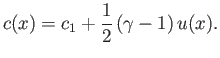(14.138)

Hence, the absolute disturbance speed in the fan is [cf., Equation (14.51)]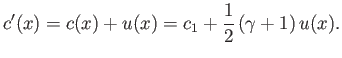(14.139)

In particular, the terminating characteristic on the left (for which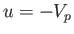) has the slope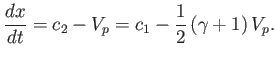(14.140)

It follows that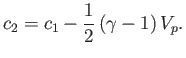(14.141)

Standard isentropic relations (see Section 14.3) then yield the density and pressure changes across the fan: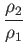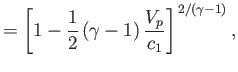(14.142)(14.143)

Here, use has been made of the fact that. At the critical piston withdrawal speed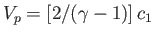, the pressure and density in regionare both reduced to zero, and the terminating characteristic on the left co-moves with the piston. Any further increase in the withdrawal speed makes no difference to the flow.

The equation of motion of the right edge of the expansion wave is(14.144)

Likewise, the equation of motion of the left edge is(14.145)

Finally, the equation of motion of a general point in the expansion wave is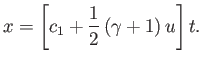(14.146)

The previous three equations can be combined to give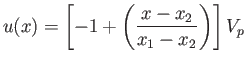(14.147)

for. In other words, the flow speed varies linearly withinside the expansion wave. It follows from Equations (14.138) and (14.141) that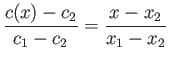(14.148)

for. Thus, the sound speed also varies linearly withinside the expansion wave. However, according to Equation (14.136),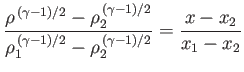(14.149)

for. Furthermore, standard isentropic relations yield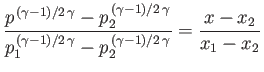(14.150)

for. Thus, neither the density nor the pressure vary linearly withinside the expansion wave.Next: Exercises Up: One-Dimensional Compressible Inviscid Flow Previous: Piston-Generated Shock Wave
Richard Fitzpatrick 2016-03-31